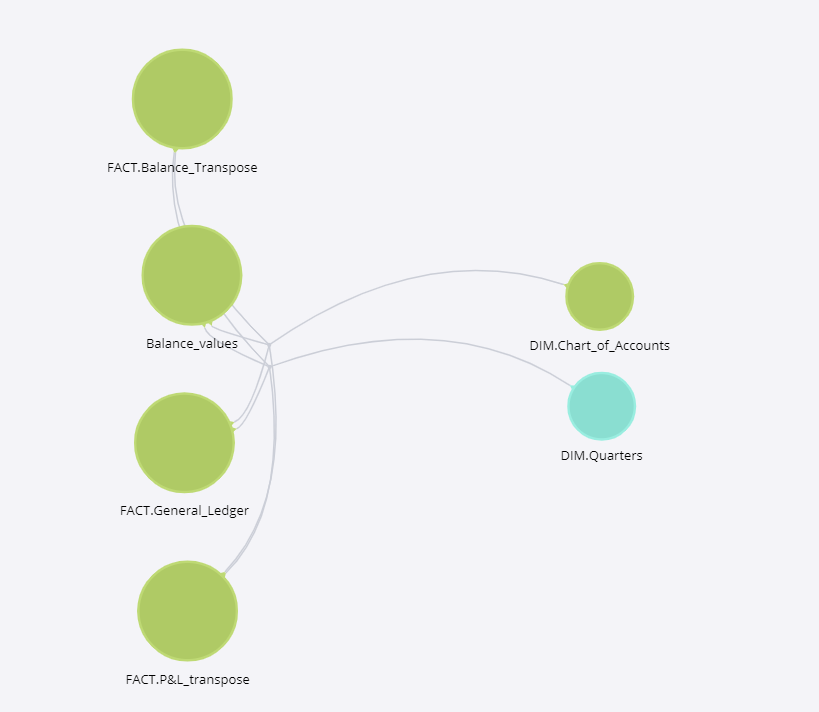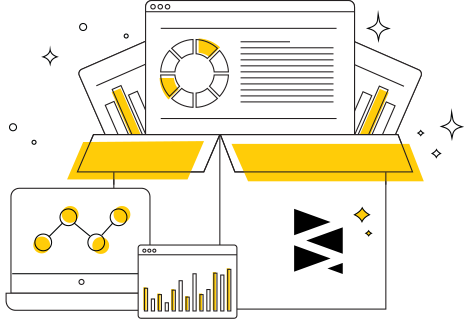# Profit & Loss Statement Dashboard

## High Level Design

### Overview

The profit and loss (P&L) statement is a financial statement that summarizes the revenues, costs, and expenses incurred during a specified period, usually a fiscal quarter or year.

These records provide information about a company's ability or inability to generate profit by increasing revenue, reducing costs or both. Some refer to the P&L statement as a statement of profit and loss, income statement, statement of operations, statement of financial results or income, earnings statement or expense statement. It`s an obligatory report in accordance to the international accounting rules as well as IFRS.

In this BI solution, we propose a method of using Sisense for forming a P&L report based on General Ledger (accounting journal entries transactions data) and analyzing P&L records aimed to cover finance performance assessment. Assessment of financial performance is primarily based on various methods of financial analysis. The aim is to achieve the desired level of complexity in evaluating firm and its activities. In the practice of financial analysis, financial ratios are mainly used for their simplicity and additional information value. It is aimed to allow employees authorized to make managerial decisions.

Data sources are commonly used and can be an official financial statement of the company Profit and Loss account and General Ledger or Trial balance of the company. It is used by a variety of stakeholders, such as credit and equity investors, the government, the public, and decision-makers within the organization. These stakeholders have different interests and apply a variety of different techniques to meet their needs.

### Goals

Prepare comprehensive finance analysis based on Profit and Loss Statement aimed to visualize these results in way enough to make managerial decision related to the finance performance management.

### Objectives

The set of the objects below enables to reach out the goal above:

• Form Profit and Loss Statements using Sisense based on General Ledger information;
• Visualize P&L structure based on main items for the reporting periods to see dynamics;
• Prepare disclose of the major information for the assessment Sales/Margin Quality Performance Analysis;
• DUPONT method of finance assessment (Returns on Equity, Sales, Assets) with ability to define trends within defined time period;
• Define EBITDA, and Profitability Ratios (GPM, OPM, EBITDA Margin) for each reporting period;
• Assess the company`s possibility to repair all obligations in dynamic in dates; (Debt Coverage Ratios);
• Estimate creditworthiness of company based on calculation debt load. Assess the possibility to serve debt and pay-off accrued interest (EBIT/Interest, EBITDA/Debt Service, Fixed Charge Coverage, Interest Paid / Average Funded Debt).

### KPIs Architecture

 Objectives KPIs Measures Data Source 1.P&L report Profit and Loss Report Profit and Loss Statement prepared under IFRS Fact.General_Ledger 2.P&L items dynamics Profit and Loss Dynamics Structure of Profit and Loss account Dynamics of major P&L items P&L_transpose 3.Sales/Margin Quality Performance Analysis General & Administrative Expense G&A expense dynamics Fact.General_Ledger EBITDA Sum(Sales)- Sum(CoS) – Sum(R&D Expense) – Sum(SG&A Expense) -Sum(Depreciation) + Sum(Other Operating Income/-Expenses) P&L_transpose Working Investments/Sales (Current Assets – Cash & Marketable Securities) / (Current Liabilities – Short Term Debt – CPLTD) Fact.General_Ledger 4. DUPONT RATIOS Return on Equity (RoE) Sum(Net Profit)/Sum(Equity) per each quartal Net Profit from Fact.P&L_transpose Equity from Fact.Balance Return on Sales (RoS) Sum(Net Profit)/Sum(Sales) per each quartal Net Profit from Fact.P&L_transpose Sales from Fact.P&L_transpose Return on Assets (RoA) Sum(NetProfit)/Sum(Assets) per each quartal Net Profit from Fact.P&L_transpose Assets from Fact.Balance_transpose 5. EBITDA & PROFITABILITY RATIOS EBITDA (earnings before interest, tax, depreciation, amortization) Sum(Sales)- Sum(CoS) – Sum(R&D Expense) – Sum(SG&A Expense) -Sum(Depreciation) + Sum(Other Operating Income/-Expenses) Sales, Cost of Sales, SG&A Expenses, Other Operating Income – from FACT.P&L_transpose GPM (Gross Profit Margin) (Sales-CoS)/Sales All from FACT.P&L_transpose EBITDA Margin (Sum(Sales)- Sum(CoS) – Sum(R&D Expense) – Sum(SG&A Expense) -Sum(Depreciation) + Sum(Other Operating Income/-Expenses)) / Sales All from FACT.P&L_transpose Operating Profit Margin Sum(Sales) – Sum(CoS) – Sum(R&D Expense) – Sum(SG&A Expense) + Sum(Other Operating Income/-Expenses)/ (Sales) All from FACT.P&L_transpose 6. Debt Coverage Ratios TOTAL DEBT/EBITDA Senior Bank Debt + Finance Lease + Subordinated Debt + Accrued interests (ST+LT) + Other Debt + Short Term Debt + Current Portion of Long Term Debt (CPLTD) / Sum(Sales)- Sum(CoS) – Sum(R&D Expense) – Sum(SG&A Expense) -Sum(Depreciation) + Sum(Other Operating Income/-Expenses) TOTAL DEBT – from FACT.Balance_transpose EBITDA from FACT.P&L_transpose NET DEBT/EBITDA (Senior Bank Debt + Finance Lease + Subordinated Debt + Accrued interests (ST+LT) + Other Debt – Cash & Marketable Securities) / (Sum(Sales)- Sum(CoS) – Sum(R&D Expense) – Sum(SG&A Expense) -Sum(Depreciation) + Sum(Other Operating Income/-Expenses)) Net Debt – from Balance EBITDA from FACT.P&L_transpose LT DEBT/EBITDA (Senior Bank Debt + Finance Lease + Subordinated Debt + Accrued interests (ST+LT) + Other Debt ) / (Sum(Sales)- Sum(CoS) – Sum(R&D Expense) – Sum(SG&A Expense) -Sum(Depreciation) + Sum(Other Operating Income/-Expenses)) LT DEBT – from Balance EBITDA from FACT.P&L_transpose 7. Creditworthiness assessment EBIT/Interest (Sum(Sales) – Sum(CoS) – Sum(R&D Expense) – Sum(SG&A Expense) + Sum(Other Operating Income/-Expenses)) /(Interest Expenses) All from FACT.P&L_Transpose EBITDA / Debt Service (Sum(Sales)- Sum(CoS) – Sum(R&D Expense) – Sum(SG&A Expense) -Sum(Depreciation) + Sum(Other Operating Income/-Expenses))/(Interest Expenses) All from FACT.P&L_Transpose Fixed Charge Coverage (Sum(Sales)- Sum(CoS) – Sum(R&D Expense) – Sum(SG&A Expense) -Sum(Depreciation) + Sum(Other Operating Income/-Expenses)) / (Interest Expenses + Lease Expense) All from FACT.P&L_Transpose Interest Paid / Average Funded Debt (Interest expenses) /(Senior Bank Debt + Finance Lease + Subordinated Debt + Accrued interests (ST+LT) + Other Debt + Short Term Debt + Current Portion of Long Term Debt (CPLTD)) Interest expenses – from FACT.P&L_transpose Others from FACT.BALANCE_transpose

### Entities Relationship Diagram### Plugins & Scripts## Implementation Kit

The following resources will enable you to design your dashboard and data model with sample data and then apply it to your own data. Note that you will need to have a previously installed version of Sisense (you can use the free trial version if you’re not a customer).